Published

# Smart Building: Household Utility Monitoring System

Use flow rate sensor to capture water consumption in household and provide visual information via web apps.

BeginnerFull instructions providedOver 3 days391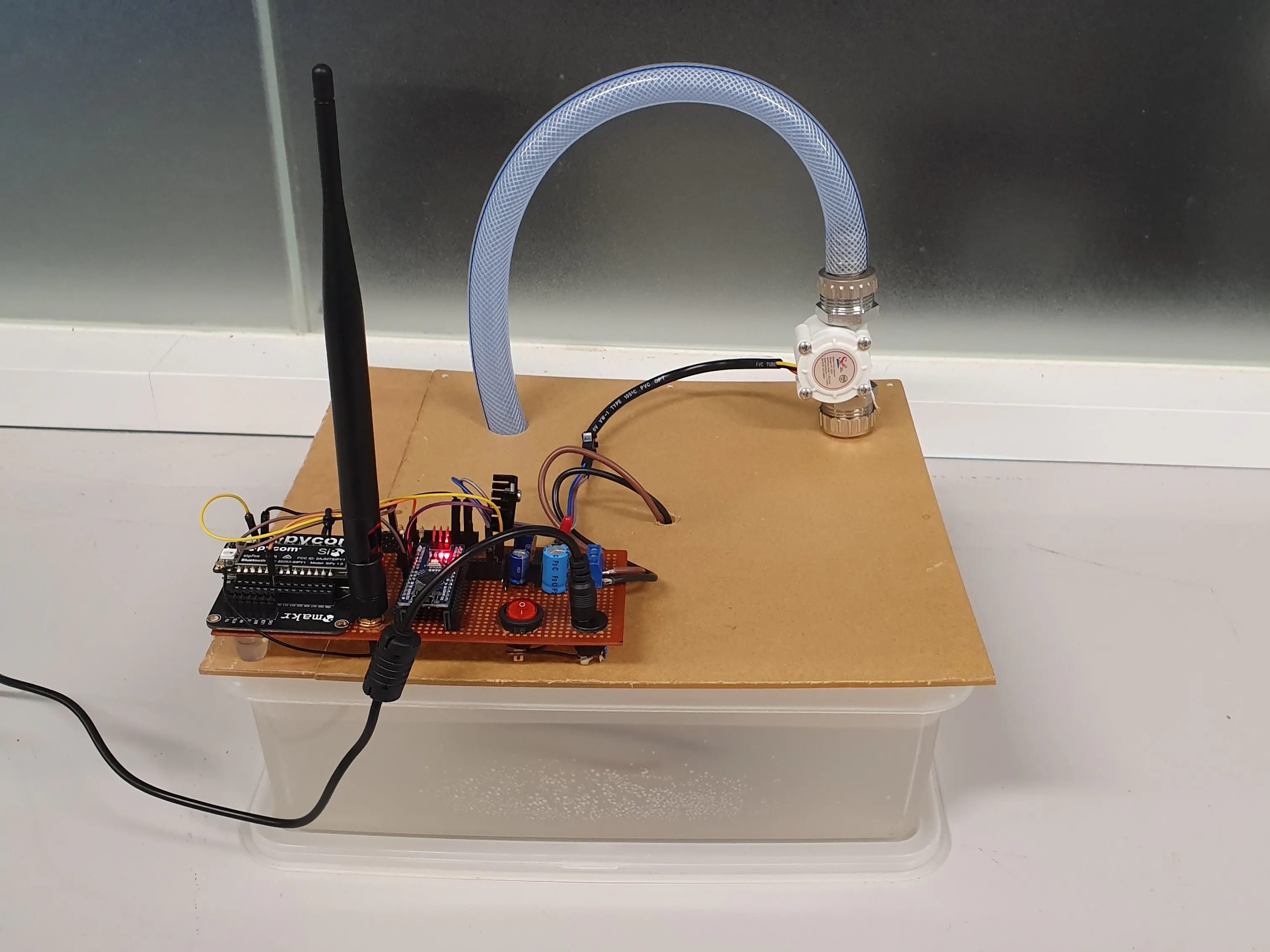## Things used in this project

### Hardware componentsArduino Nano R3
×1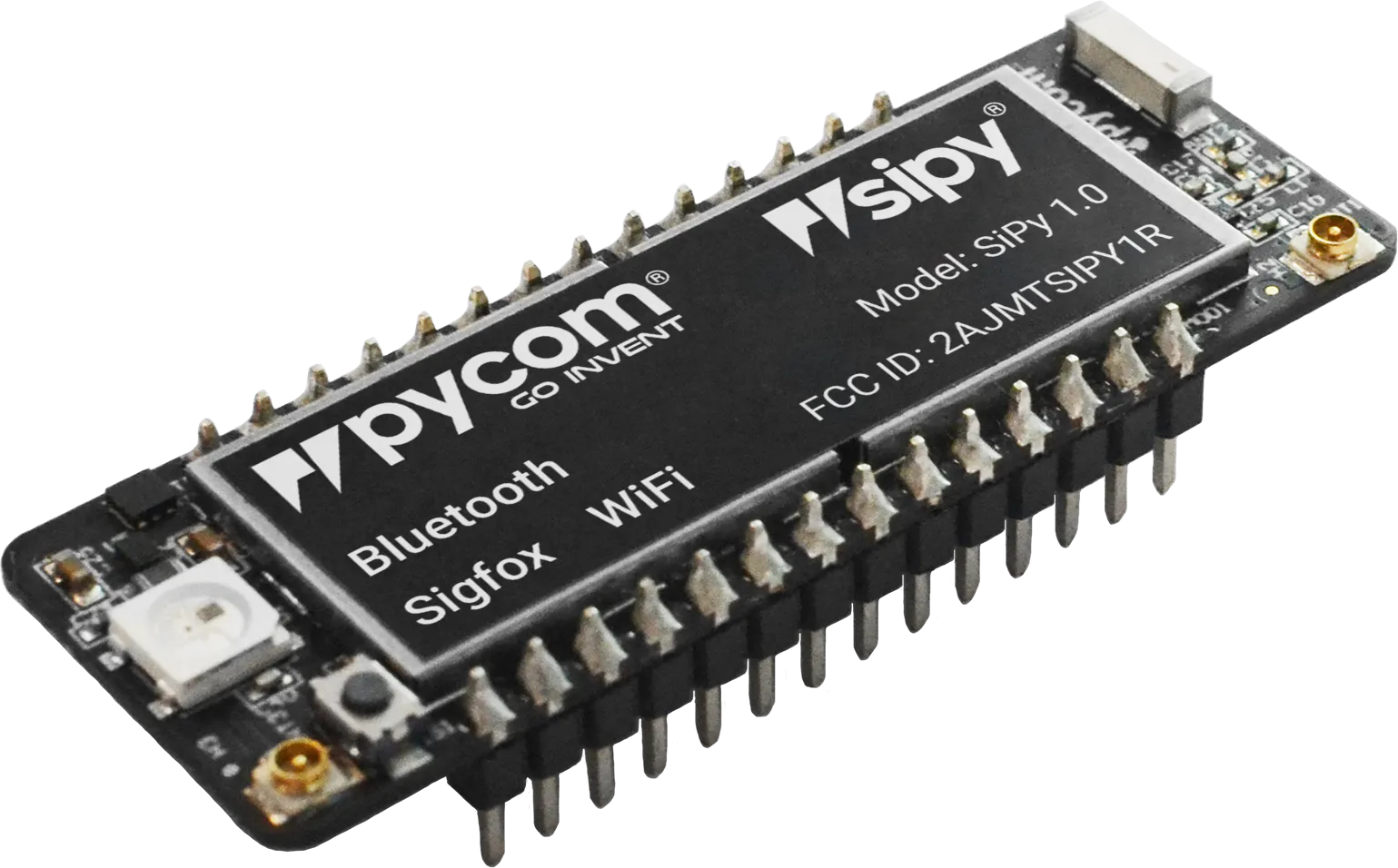Pycom SiPy
×1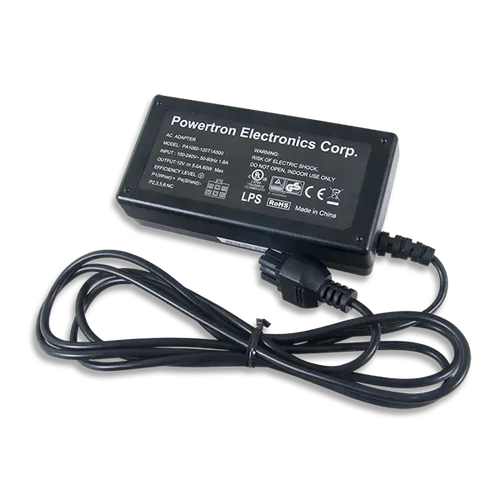Digilent 60W PCIe 12V 5A Power Supply
×1
 Bilge Pump 12V 360GPH
×1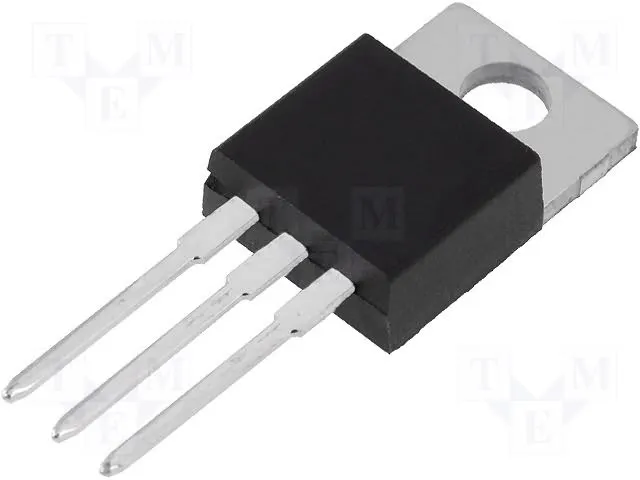Linear Regulator (7805)
×1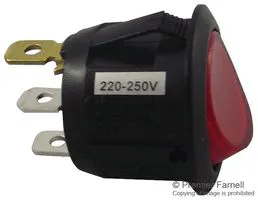Rocker Switch, Splash Proof
×1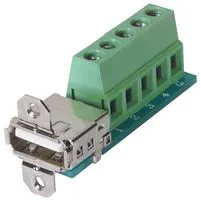Terminal Block Interface, Terminal Block
×1Jumper wires (generic)
×20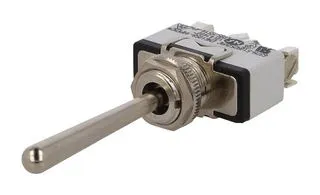Toggle Switch, Metal Lever
×1
 StripBoard (Veroboard)
×1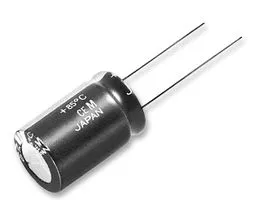Capacitor 470 µF
×1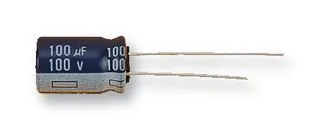Capacitor 100 µF
×2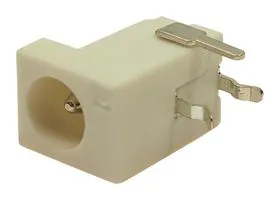DC Power Connector, Socket
×1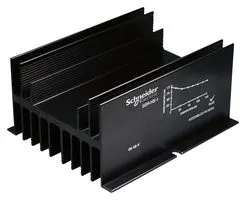Heat Sink, Panel
×1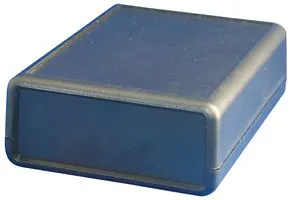Plastic Enclosure, Hand-Held Plastic Box Style 2
×1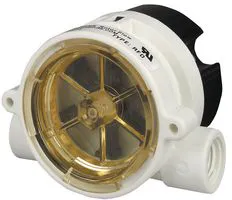Flow Sensor, Pulsed Output
×1

### Software apps and online services

 Pycom Visual Studio Code (Pymakr)Arduino IDEThingSpeak API

### Hand tools and fabrication machinesDrill / Driver, Cordless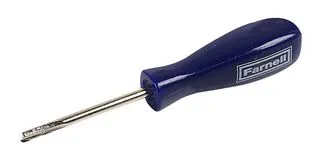Track Cutter, StripboardSoldering iron (generic)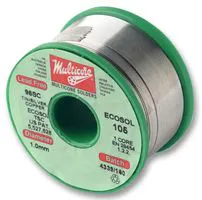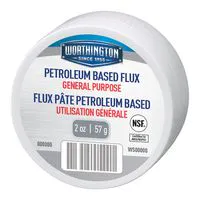Solder Flux, Soldering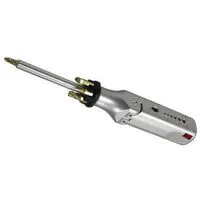Multitool, Screwdriver

## Custom parts and enclosures

### EnclosurePhoto2

Overall view of the prototype without pumps controller circuitry and power source from 9V rechargeable battery 800mAH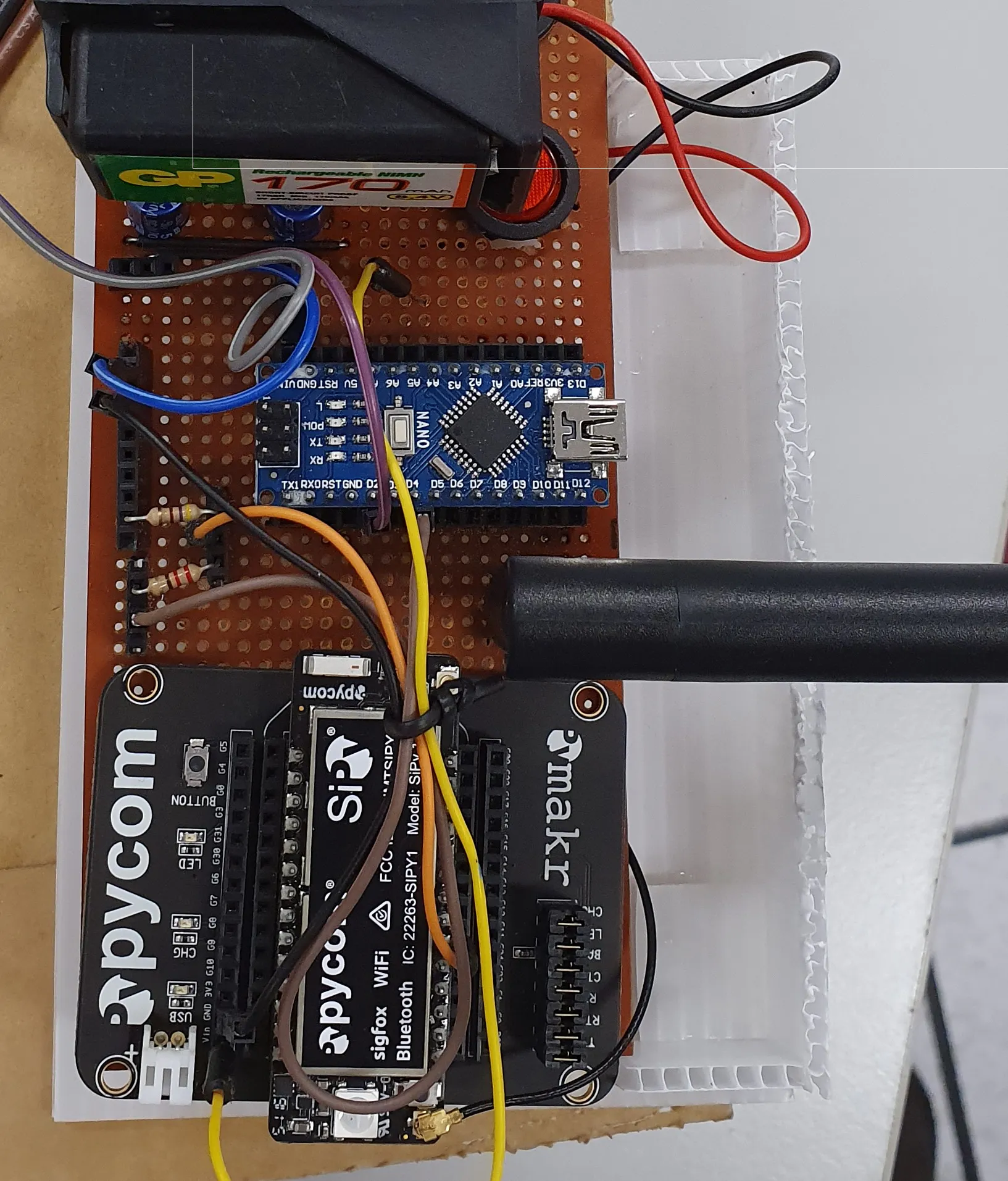### EnclosurePhoto1

External view of the prototype appearance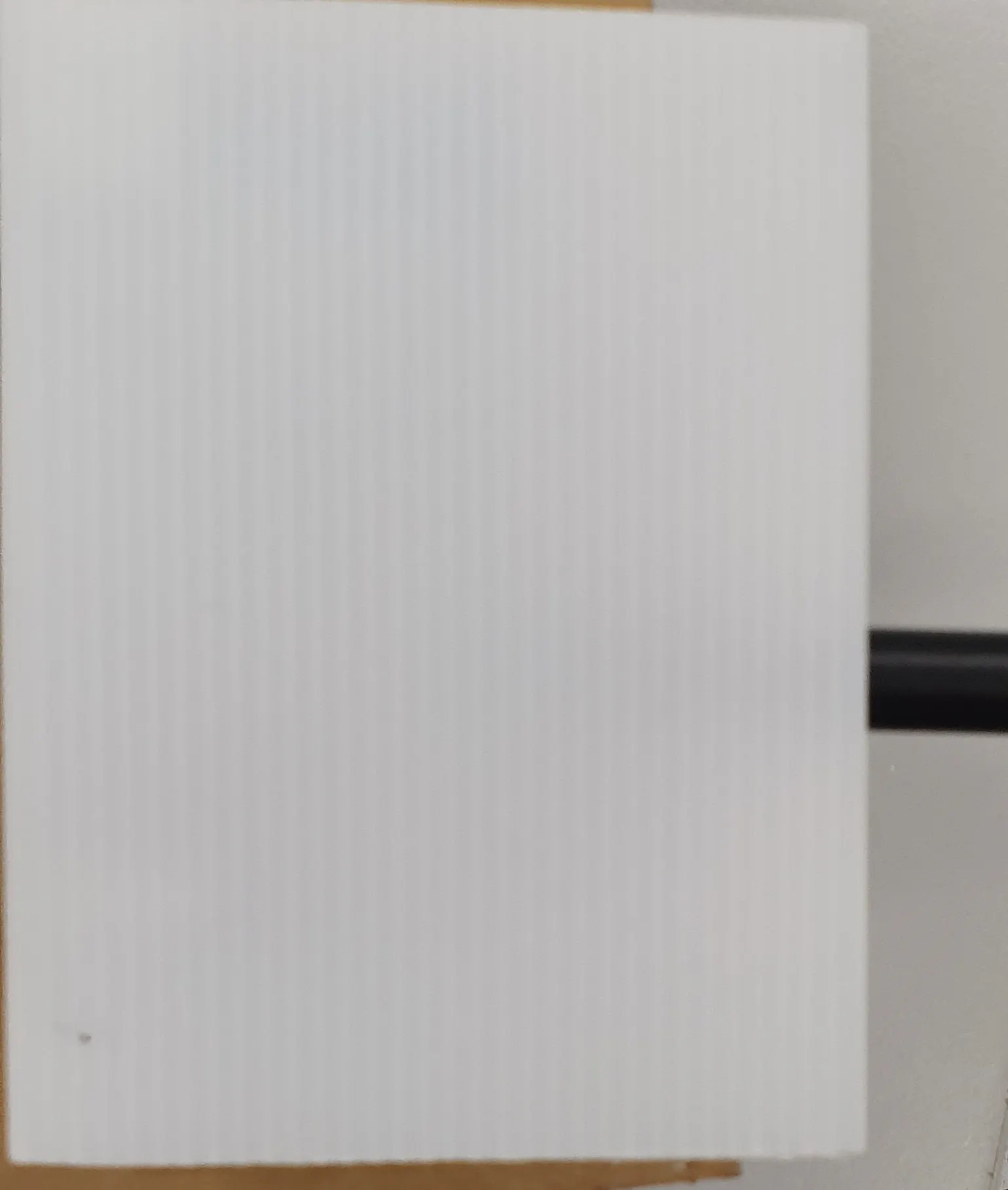### Prototype Appearance

Appearance Drawing (Not to Scale)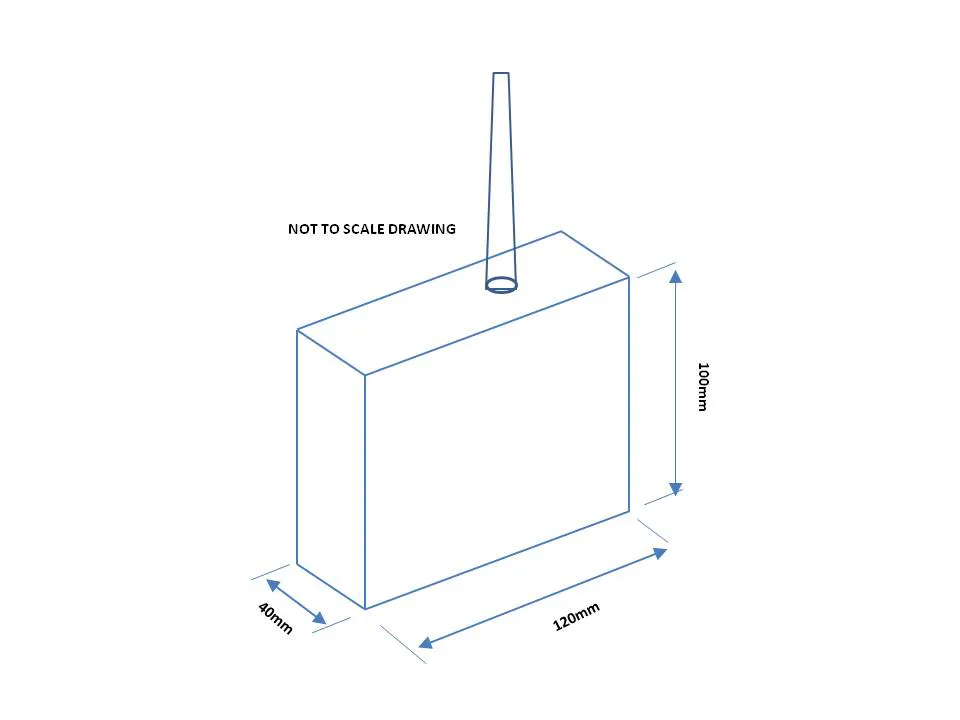## Schematics

### Arduino Communication

This is a Diagram showing the communication between the Pycom and the Arduino Uno.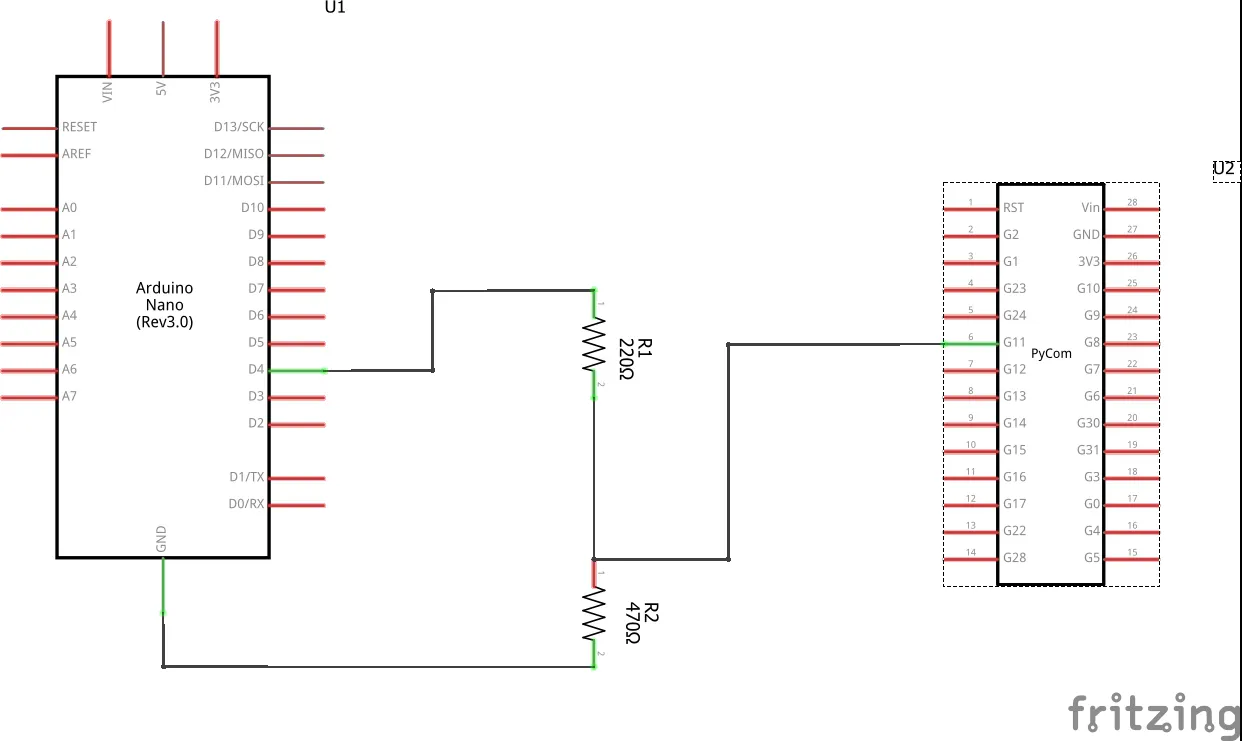### Power Supply Schematic

This is showing the Schematic Diagram of the power supply.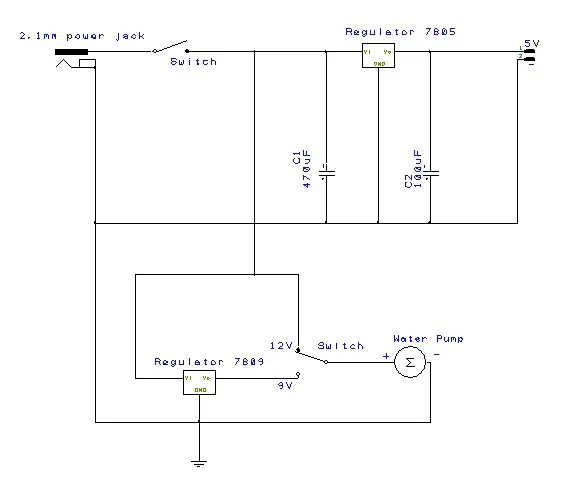### Water Flow Rate Sensor

This is a Diagram showing how the Water Flow rate sensor has been connected.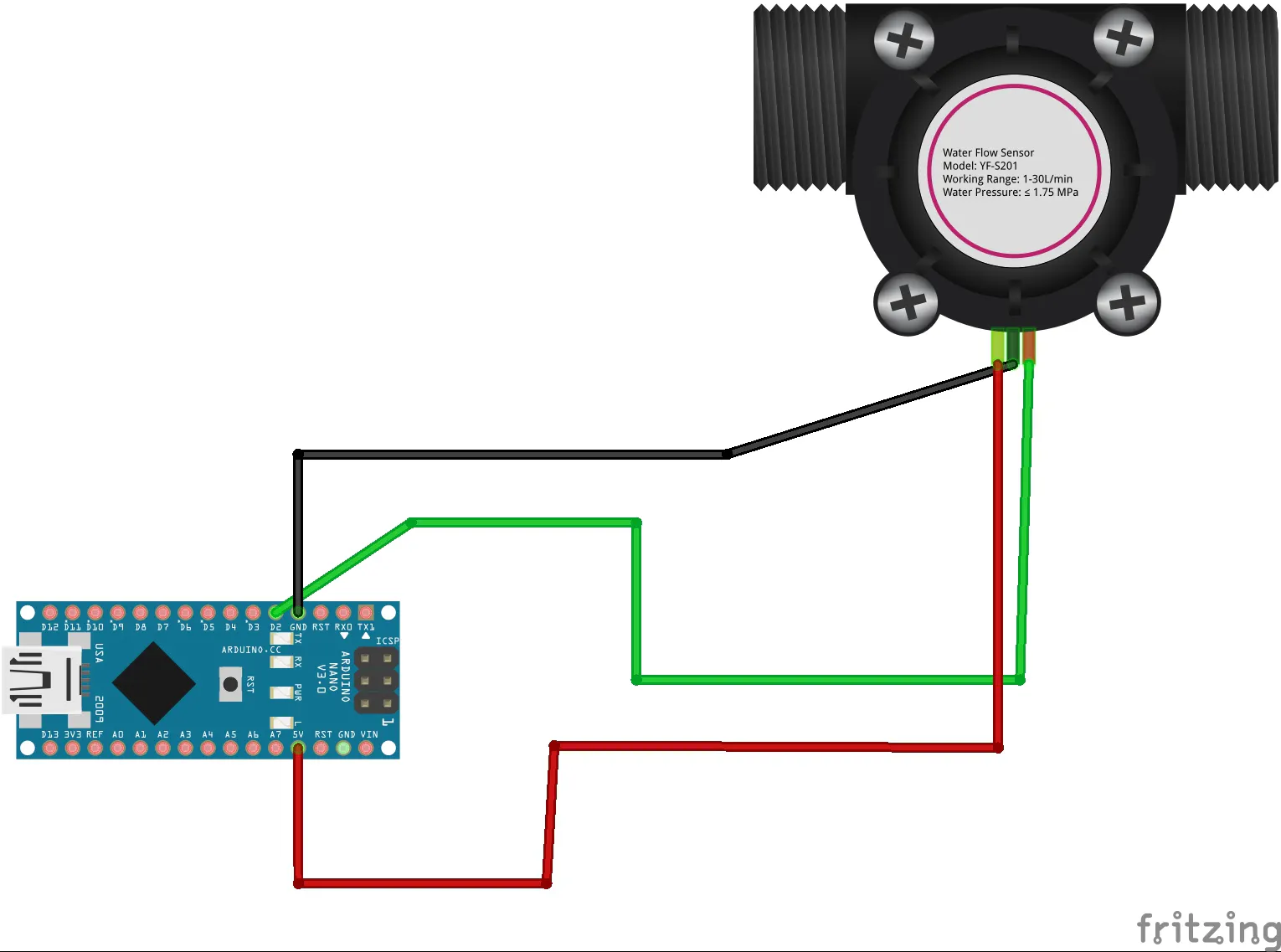## Code

### Thinkspeak_MatLab_Code_FlowRate

MATLAB
This coding is used to recombine HighByte & LowByte to form decimal value in Thinkspeak visual display.
```% Read flowrate_H and flowrate_L and total consumption from a public ThingSpeak channel created

% Channel ID to read data from

% Obtain the channel ID and write API key for your channel
% Replace [] with your channel ID
writeChID = 890527;
% Enter your Write API Key between the ''
writeAPIKey = '1VNEDKGLY4QK417D';

% Read air temperature, time stamps, and wind speed from weather station

% Calculate and display flowrate data
Flowrate = Flowrate_H + (Flowrate_L * 0.1);
display(Flowrate,'FlowRate ');

% Calculate and display total consumption data
Total_Consumption = Total_Consumption_H + (Total_Consumption_L * 0.01);
display(Total_Consumption,'Total_Consumption');

% Calculate billing
Total_Billing = (Total_Consumption *0.001) * 2.7
display(Total_Billing,'Total Billing (S\$)');

% Plot flowrate and total_consumtion to your channel
thingSpeakWrite(writeChID,[Flowrate,Total_Consumption,Total_Billing],'Fields',[1,2,3],...
'TimeStamps',time,'WriteKey',writeAPIKey);
```

### FlowMeterDIY_JLa_sigfox.ino

Arduino
Programming Code for Arduino
```/*
Liquid flow rate sensor -DIYhacking.com Arvind Sanjeev

Measure the liquid/water flow rate using this code.
Connect Vcc and Gnd of sensor to arduino, and the
signal line to arduino digital pin 2.

*/
#include <SoftwareSerial.h>

SoftwareSerial nanoSend(3,4); //Rx,Tx

byte statusLed    = 13;

byte sensorInterrupt = 0;  // 0 = digital pin 2
byte sensorPin       = 2;

// The hall-effect flow sensor outputs approximately 4.5 pulses per second per
// litre/minute of flow.
float calibrationFactor = 4.5;

volatile byte pulseCount;

float flowRate;
unsigned int frac;
unsigned int flowMilliLitres;
//unsigned long totalMilliLitres;
float totalMilliLitres;
unsigned long oldTime;
unsigned long oldTime1;

void setup()
{

// Initialize a serial connection for reporting values to the host
Serial.begin(9600);
nanoSend.begin(9600);
// Set up the status LED line as an output
pinMode(statusLed, OUTPUT);
digitalWrite(statusLed, HIGH);  // We have an active-low LED attached

pinMode(sensorPin, INPUT);
digitalWrite(sensorPin, HIGH);

pulseCount        = 0;
flowRate          = 0.0;
flowMilliLitres   = 0;
totalMilliLitres  = 0;
oldTime           = 0;

// The Hall-effect sensor is connected to pin 2 which uses interrupt 0.
// Configured to trigger on a FALLING state change (transition from HIGH
// state to LOW state)
attachInterrupt(sensorInterrupt, pulseCounter, FALLING);
}

/**
* Main program loop
*/
void loop()
{

if((millis() - oldTime) > 5000)    // Only process counters once per second
{
// Disable the interrupt while calculating flow rate and sending the value to
// the host
detachInterrupt(sensorInterrupt);

// Because this loop may not complete in exactly 1 second intervals we calculate
// the number of milliseconds that have passed since the last execution and use
// that to scale the output. We also apply the calibrationFactor to scale the output
// based on the number of pulses per second per units of measure (litres/minute in
// this case) coming from the sensor.
flowRate = ((1000.0 / (millis() - oldTime)) * pulseCount) / calibrationFactor;

// Note the time this processing pass was executed. Note that because we've
// disabled interrupts the millis() function won't actually be incrementing right
// at this point, but it will still return the value it was set to just before
// interrupts went away.

// Divide the flow rate in litres/minute by 60 to determine how many litres have
// passed through the sensor in this 1 second interval, then multiply by 1000 to
// convert to millilitres.
flowMilliLitres = (flowRate / 60)* 1000;

// Add the millilitres passed in this second to the cumulative total
totalMilliLitres += (flowMilliLitres)* 0.001;

// Print the flow rate for this second in litres / minute
Serial.print("Flow rate: ");
Serial.print(int(flowRate));  // Print the integer part of the variable
Serial.print(".");             // Print the decimal point
// Determine the fractional part. The 10 multiplier gives us 1 decimal place.
frac = (flowRate - int(flowRate)) * 10;
Serial.print(frac, DEC) ;      // Print the fractional part of the variable
Serial.print("L/min");
// Print the number of litres flowed in this second
/*Serial.print("  Current Liquid Flowing: ");             // Output separator
Serial.print(flowMilliLitres);
Serial.print("mL/Sec");*/

// Print the cumulative total of litres flowed since starting
Serial.print("  Output Liquid Quantity: ");             // Output separator
Serial.print(totalMilliLitres);
Serial.println("L");

// Reset the pulse counter so we can start incrementing again
pulseCount = 0;

// Enable the interrupt again now that we've finished sending output
attachInterrupt(sensorInterrupt, pulseCounter, FALLING);
oldTime = millis();
}

if((millis() - oldTime1) > 120000)
{
byte flowrate_H = int(flowRate);
byte flowrate_L = frac;
byte totalML_H = floor(totalMilliLitres);
byte totalML_L = (totalMilliLitres - totalML_H) * 100;

String dataMsg = "";

dataMsg += flowrate_H;
dataMsg += ",";
dataMsg += flowrate_L;
dataMsg += ",";
dataMsg += totalML_H;
dataMsg += ",";
dataMsg += totalML_L;

Serial.print("\n dataMsg : ");
Serial.println(dataMsg);
nanoSend.print(dataMsg);
oldTime1 = millis();
}
}

/*
Insterrupt Service Routine
*/
void pulseCounter()
{
// Increment the pulse counter
pulseCount++;
}
```

## Credits

### Tan Wei Kang

1 project • 0 followers
Student of ITE College Central Singapore